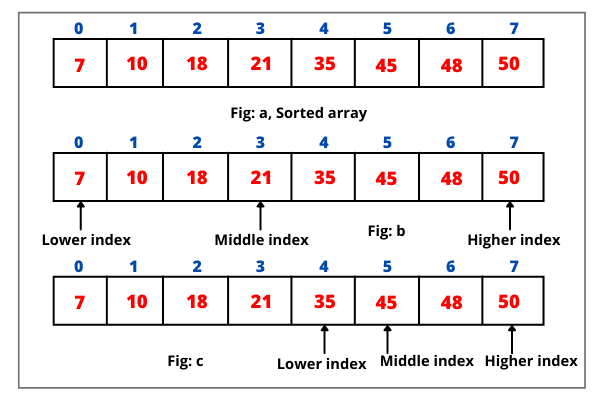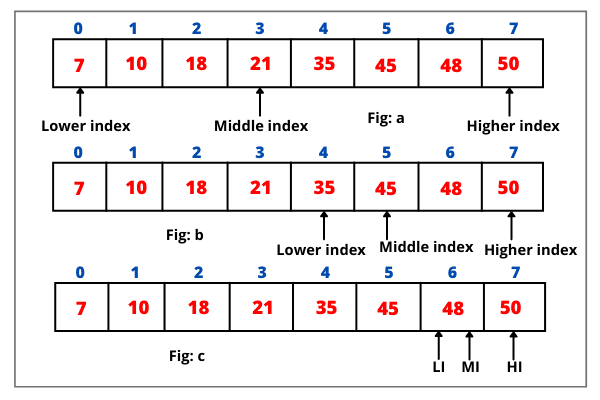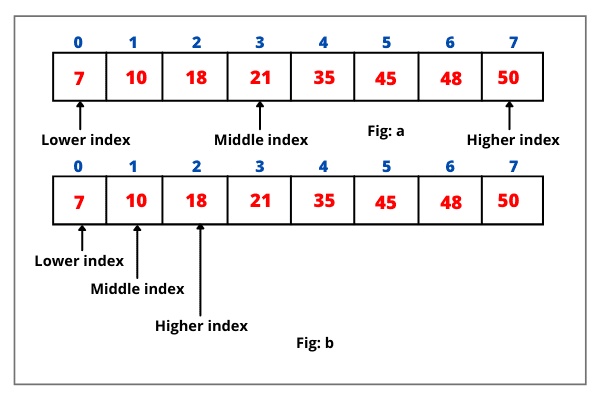# Binary Search in Java for Sorted Array

The binary search in java is the most efficient search algorithm than linear search for finding an element in the sorted array.

It is a new technique to search an element in the minimum possible time because binary search is so much faster than linear search.

The binary search in java always works on the principle of sorting array which means array elements available in binary search must be in ascending or descending order.

In the binary search technique, the searching element is always compared with the middle element of the sorted array.

If the array is not sorted then first sort the array either ascending or descending order and then perform binary search algorithm.

For the sake of simplicity in the discussion, we assume that the elements in the array are already sorted in ascending order.

## How does Binary Search Algorithm work in Java for sorted Array?

To search an array x for key, the binary search first compares the key with the element situated in the middle of the array (say x[mid]).

Consider the following three cases:

1. If the key is less than the middle element (i.e. key < x[mid]), then x[mid] and all elements greater than x[mid] are eliminated from the search.

2. If the key is equal to the middle element (i.e. key = x[mid]), the search ends with a match successfully.

3. If the key is greater than the middle element (i.e. key > x[mid]), then x[mid], and all elements less than x[mid] can be eliminated.

Clearly, after examining a single location, the binary search method eliminates at least half of the elements in the array from search after each comparison.

Let’s understand the binary search algorithm with the help of various examples.

Example 1: Consider the following sorted array below:Let the search element (i.e. key) be 45. Here, the lower index is 0, and the higher index is 7. Thus, the middle index is (0 + 7)/2 = 3. Look at the above figure b.

Binary search first compares 45 to the middle element of the array, which is 21. Since the search element 45 is greater than the element situated at the middle index and the array is sorted in ascending order, therefore, the “sub-array” [7, 10, 18, 21] is eliminated from any further searching.

If search element > middle element, set the lower index to middle index + 1.

If search element < middle element, set the higher index to middle index – 1.

If search element == middle element, a match is found and returns the middle index.

Since the search element is greater than middle element, the lower index is changed to the middle index + 1, as shown in the below figure c.

Thus, the lower index is now 4. After first comparison, the middle index is now (4 + 7)/2 = 5.

Now you can observe in the above figure, the search element and the element situated at the middle index are the same. Therefore, Java binary search algorithm stops by returning the middle index, 5.

Example 2:

Let’s take another sorted array example. Suppose the search element is 56. The lower index is 0 and the higher index is 7. So, the middle index is (0 + 7)/2 = 3.

Now binary search compares 56 to the middle element (which is 21) of the array. Since the search element 56 is greater than the element at middle index, therefore, the “sub-array” [7, 10, 18, 21] has been eliminated from any further searching.

Since the search element is greater than the middle element, the lower index is changed to the middle index + 1, as shown in the below figure b.Thus, the lower index is now 4. After first comparison, the middle index is now (4 + 7)/2 = 5.

Now you can observe, once again the search element is greater than the element at the middle index, therefore, the lower index is again changed to middle index + 1.

Now, the lower index is 6 and the middle index is (6 + 7)/2 = 6, as shown in the above figure.

Again, search element 56 is greater than the element at middle index 6. The lower index is again changed to middle index + 1. Therefore, now, the lower index is 7 and the middle index is (7 + 7)/2 = 7.

Now you can observe that the search element is larger than the element present at the middle index 7. Therefore, the lower index becomes middle index + 1 that is now 8.

Since the lower index 8 is greater than higher index 7, the search space is zero. In this case, the binary search algorithm ends with the conclusion that the search element 56 is not present in the array.

Example 3:

Consider a sorted array below as shown in the figure.Let the search element be 15. The lower index is 0 and the higher index is 7. Thus, the middle index is (0 + 7)/2 = 3.

The search element 15 is smaller than the element present at the middle index. The higher index is changed to middle index – 1. Thus, the higher index is 2 and the middle index is (0 + 2)/2 = 1.

The search element 15 is greater than 10, element present at the middle index. Thus, the lower index is changed to middle index + 1.

Now, the lower index will become 2 and the middle index become (2 + 2)/2 = 2.

Observe that the search element 15 is smaller than the element at the middle index. Therefore, the higher index will be middle index – 1, that is 1.

Since the higher index is smaller than the lower index, therefore, the search space is zero. In this case, the binary search algorithm stops the search with the conclusion that the search item is not present in the array list.

## Example Program based on Java Binary Search Array

1. Let’s take a very simple example program where we will create a sorted array and check whether a particular element is present in the array or not using binary search method. We will also display the index location of search element in the array.

Program code:

```package arraysProgram;
public class BinarySearch
{
public static int search(int[] arr, int n, int key)
{
int loIndex = 0; // Lowest index of the array.
int hiIndex = n - 1; // Highest index.
int midIndex; // Middle index.

while(hiIndex >= loIndex)
{
midIndex = (hiIndex + loIndex)/2; //  Getting the middle index.
if(key == arr[midIndex])
return midIndex; // key found and exits from array.
if(key < arr[midIndex])
hiIndex = midIndex - 1;
else
loIndex = midIndex + 1;
}
}
public static void main(String[] args)
{
int[ ] arr = {7, 10, 18, 21, 35, 45, 48, 50};
int i = BinarySearch.search(arr, 7, 45); // returns 5.
System.out.println("Element found in the array at the index location " +i);

int j = BinarySearch.search(arr, 7, 56); // returns -1.
int k = BinarySearch.search(arr, 7, 15); // returns -1.
int l = BinarySearch.search(arr, 7, 10); // returns 1.
System.out.println("Element found in the array at the index location " +l);
}
}
```
```Output:
Element found in the array at the index location 5
Element found in the array at the index location 1
```

2. Let’s take another example based on the binary search algorithm where we will take all the inputs from the user and will check whether the element is present in the array or not.

We will also find the index location of the search element. In this program, we will use Scanner class for taking input from the keyboard.

Program code:

```package arraysProgram;
import java.util.Scanner;
public class BinarySearch
{
public static int search(int[ ] arr, int key)
{
int loIndex = 0; // Lowest index of the array.
int hiIndex = arr.length - 1; // Highest index.
int midIndex; // Middle index.

while(hiIndex >= loIndex)
{
midIndex = (hiIndex + loIndex)/2; //  Getting the middle index.
if(key == arr[midIndex])
return midIndex; // key found and exits from array.
if(key < arr[midIndex])
hiIndex = midIndex - 1;
else
loIndex = midIndex + 1;
}
}
public static void main(String[] args)
{
Scanner sc = new Scanner(System.in);
int[ ] arr = new int;
System.out.println("Enter 10 numbers in ascending order: ");
for(int i = 0; i < 10; i++)
{
arr[i] = sc.nextInt();
}
System.out.println("Enter a number that has to be searched: ");
int key = sc.nextInt();
int i = BinarySearch.search(arr, key);
System.out.println(i);
}
}
```
```Output:
Enter 10 numbers in ascending order:
2
4
6
8
10
15
20
25
30
45
Enter a number that has to be searched:
60
-1

```

## Why binary search in Java has come into existence if we already linear search algorithm available?

As we know that linear search algorithm is slow. That’s why, binary search algorithm has come into existence in Java.

A binary search algorithm is much faster than linear search for a large array size. Let’s understand it with the help of a simple scenario.

Let us assume two cases:

Scenario 1:

Suppose we have 10 items in the array and we have to check whether a specific item is present in the array or not.

In this case, we can use the linear search technique because searching 10 items are very much easier and it will also not affect the performance of the system. So, we will not feel the slowness in our system performance.

Scenario 2:

Suppose we have to search an element between 1 to 50 thousand. Assume that the number (i.e. element) that is searching is present at the last index location of the array.

Now think that if we will use linear search algorithm in this case, how much time it will take to move from the 0th index location to last index location.

Of course, linear search will take a huge amount of time. To overcome this problem, Java language introduced the concepts of the binary search algorithm.

Hope that this tutorial has covered almost all the important points related to the binary search algorithm in java for sorted array with example programs. I hope that you will have understood the basic concepts of binary search in java using array.